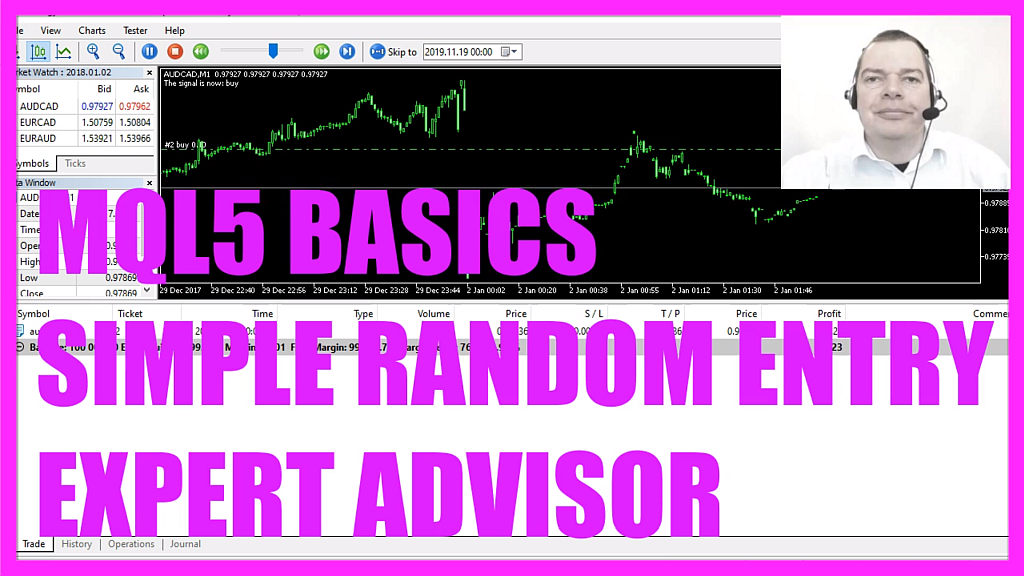In this video we are going to trade random entries, you might find random entries very strange but I promise that you might find the results very interesting, so let’s find out how to do that with mql5.
To get started please click on the little icon here or press F4 on your keyboard, now you should see the Metaeditor window and here you want to click on file, new file, expert advisor from template, continue, I will call this file simple random entry ea, click on continue, continue and finish.
Now you can delete everything above the on tick function and the two comment lines here, we start with an include function to include the file trade dot mqh, this one comes with simplified trading functions. Now we create an instance of ctrade that will be called trade and in the next step we will create an input variable that will be an integer variable called mass test and the first initial value is one, that one will become interesting in the next video when we talk about mass testing.
Inside of the on tick function we want to calculate the ask price that is done by using symbol info double for the current symbol on the chart, we use symbol underscore ask – all in capital letters – to calculate the ask price and with normalized double and underscore digits we are going to automatically calculate the right number of digits behind the dot that depends on the currency pair we use because some currency pairs have three digits behind the dot while others have five digits behind the dot.
Let’s repeat that process, this time we want to calculate the bid price, everything is the same except of this parameter, this time we are using symbol underscore bid – all in capital letters – and for the signal we create a string variable that is called signal, so far we have no value because that’s what we are going to calculate now.
Our random generator needs an initial value and we use get tick count to do that, get tick count returns the number of milliseconds that have passed since the system was started, math srand sets the starting point for the pseudorandom integers, so let’s actually calculate a random number by using math rand and this expression here will create either zero or a one and when the random number equals zero we want to set our signal to buy, otherwise, if the random number equals one our signal should have the value sell because now we want to sell.
So if the signal equals sell and positions total is below one – that would mean we have a sell signal and no open positions – and now we use trade dot sell to sell ten micro lot.